# 3.19: Solutions to Selected Problems, Part A

Problem CO1.1.

The HO-C=O or CO2H group present in all of the amino acids is called a carboxylic acid.

An additional carboxylic acid is present in aspartic acid and glutamic acid.

The H2N-C=O or CONH2 group present in asparagine and glutamine is called an amide.

Problem CO1.2.

a) aldehyde b) ketone c) ketone d) aldehyde

Problem CO1.3.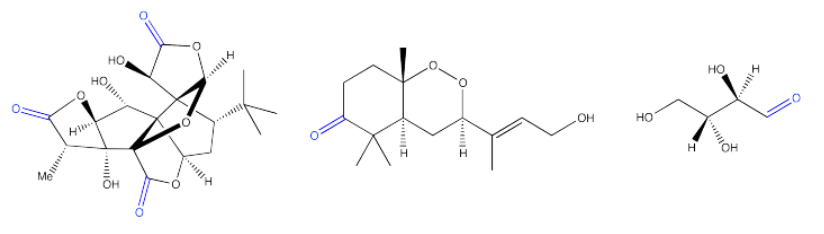Figure $$\PageIndex{1}$$:Figure $$\PageIndex{2}$$:

a) The double bond means two pairs of electrons are shared between the carbon and oxygen, instead of just one. As a result, the oxygen is able to pull more electron density away from the carbon. The carbon becomes much more positive in this case than in the case of a double bond.

Not only that, but the second bond between the carbon and the oxygen is a pi bond. Those electrons are farther from the nucleus than a sigma bond, in which the electrons are tightly held between the atoms. That means the pi electrons are more easily drawn toward the oxygen, so the bond becomes even more polarized.

b) A C=N bond would be very similar to a C=O bond, because nitrogen is the third most electronegative element after oxygen and fluorine (ignoring noble gases).Figure $$\PageIndex{3}$$:Figure $$\PageIndex{4}$$:Figure $$\PageIndex{5}$$: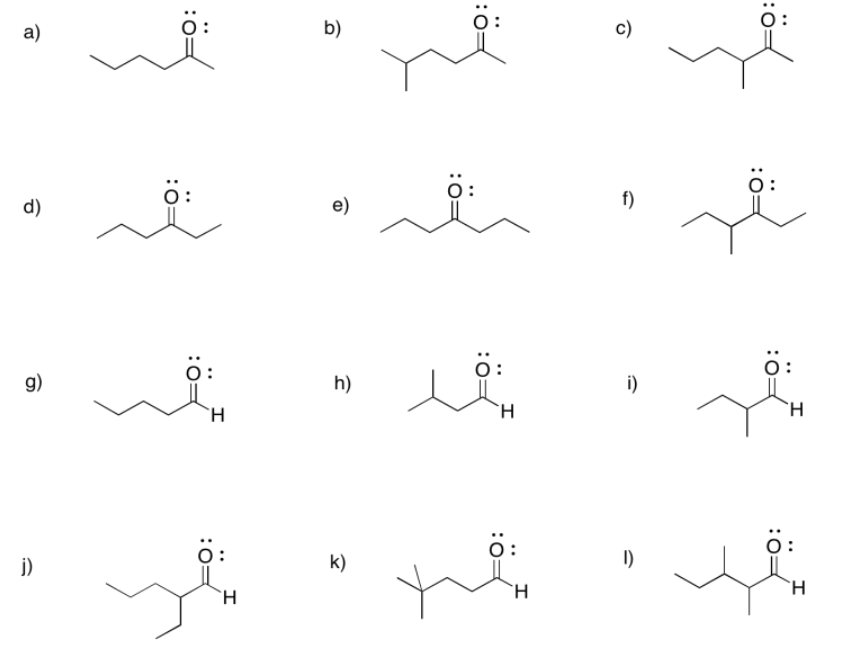Figure $$\PageIndex{6}$$:Figure $$\PageIndex{7}$$:

a) 2-hexanone or hexan-2-one b) 5-methylhexan-2-one c) 3-methylhexan-2-one

d) hexan-3-one e) heptan-4-one f) 4-methylhexan-3-one

g) hexanal h) 3-methylbutanal i) 2-methylbutanal

j) 2-ethylpentanal k) 4,4-dimethylpentanal l) 2,3-dimethylpentanal

m) 3-ethoxypropanal n) 4-chloropentan-2-one o) 4-aminohexan-3-one

p) hex-4-en-2-one (in the solution above, (E)-hex-4-en-2-one is shown first and (Z)-hex-4-en-2-one is shown second).

q) hept-4-yn-2-one r) benzaldehyde (this is a common name adopted for formal naming. Benz means a carbon attached to a benzene ring.)Figure $$\PageIndex{8}$$: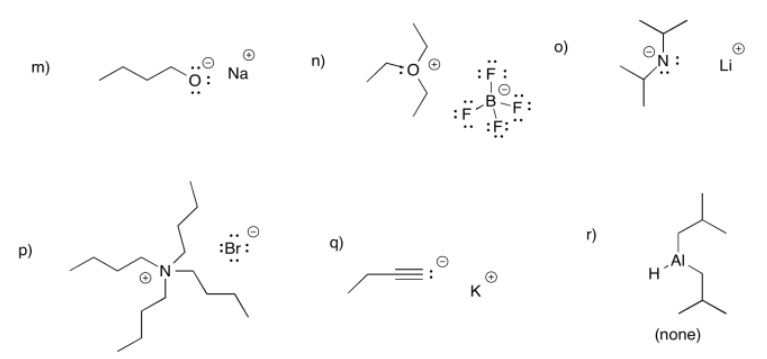Figure $$\PageIndex{9}$$:Figure $$\PageIndex{10}$$: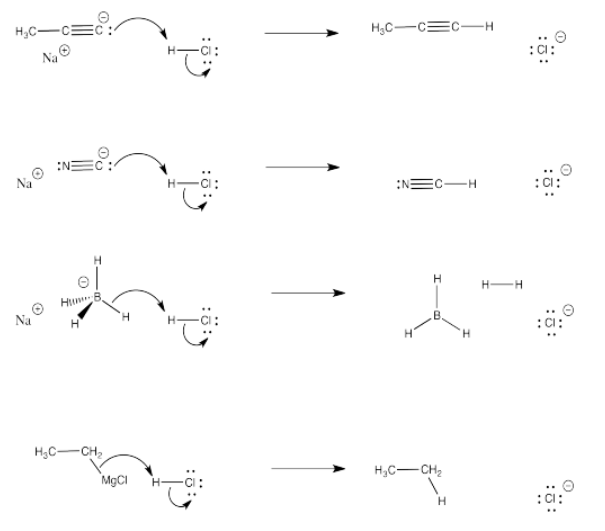Figure $$\PageIndex{11}$$:Figure $$\PageIndex{12}$$:Figure $$\PageIndex{13}$$:Figure $$\PageIndex{14}$$:Figure $$\PageIndex{15}$$:

The LUMO in this case is the π*, an antibonding level. If electrons populate this level, the π bond will break.Figure $$\PageIndex{16}$$:

Both the imine (b) and nitrile (c) have a low-lying pi antibonding level (π*), similar to a carbonyl.

1. On the basis of steric crowding, the first one is most reactive, then the last one, then the middle.
2. On the basis of steric crowding, the last one is most reactive, then the first one, and then the middle.

Although there is a large group on the nitrogen in that last compound, the site of reactivity is the carbon, which is less crowded.

c) On the basis of electronics, the middle one is most reactive, then the last, and then the first. The fluorine atom is very electronegative and pulls electron density towards itself. That leaves more positive charge on the nearby carbonyl carbon. The more fluorines on that nearby carbon, the more positive the carbon. The more positive the carbon, the more it attracts electrons from a nucleophile.

a) propanal b) butanal c) propanal, again!

d) pentanal e) hexanal f) heptanal

a) 3-pentanone b) 3-hexanone c) 4-heptanone

d) 2-butanone e) 3-octanone f) 5-decanone

a) 2-methyl-3-pentanone or 2-methylpentan-3-one b) 4-ethyl-3-hexanone or 4-ethylhexan-3-one

c) 3,3-dimethyl-2-butanone or 3,3-dimethylbutan-2-one d) 2,5,5-trimethyl-4-heptanone or 2,5,5-trimethylheptan-4-one

e) 6-ethyl-4-methyl-3-octanone or 6-ethyl-4-methyloctan-3-one f) 6-ethyl-4,5-dimethyl-3-octanone or 6-ethyl-4,5-dimethyloctan-3-oneFigure $$\PageIndex{17}$$:Figure $$\PageIndex{18}$$:Figure $$\PageIndex{19}$$:Figure $$\PageIndex{20}$$:

Ammonium chloride has an N-H bond, normally less polar than the O-H bond of water. However, the positive charge makes this compound give up a proton more easily, because it results in a neutral (uncharged) ammonia molecule.

Sodium carbonate has a polar O-H bond, just like water. However, the anion that results from loss of a proton is resonance-stabilised. That makes this compound more acidic than water.Figure $$\PageIndex{21}$$:Figure $$\PageIndex{22}$$:Figure $$\PageIndex{23}$$:Figure $$\PageIndex{24}$$:Figure $$\PageIndex{25}$$:

a) In acetylide, the lone pair is on a linear carbon or sp carbon. In methyl anion, the lone pair is on a tetrahedral carbon or sp3 carbon. The description "sp" indicates that sigma bonding to neighbors involves a 2s orbital and a 2p orbital on carbon; there is a 50% contribution from the s orbital.

The description "sp3", on the other hand, indicates that sigma bonding to neighbors involves a 2s orbital and three 2p orbitals; there is a 25% contribution from the s orbital.

The 2s orbital is lower in energy than the 2p orbital. The greater the s orbital contribution to the bond (or in this case to the lone pair), the lower it is in energy. Thus, a lone pair on an sp carbon is lower in enrgy than a lone pair on an sp3 carbon.

b) In cyanide, the same argument outlined in pary (a) hold true. In addition, the nearby electronegative nitrogen stabilizes the charge by drawing electron density toward itself.

1. CH3OK, because of the ionic O-K bond. This is an anionic nucleophile. It is more reactive and nucleophilic than the corresponding neutral nucleophile.
2. CH3NH2, because nitrogen is less electronegative than oxygen. Its lone pair is held less tightly and is more easily donated to the electrophile.
3. NaCCH, because the neighbouring carbon in this case does not have the inductive electron-withdrawing effect that the nitrogen does in the case of NaCN. In that case, the lone pair is stabilized and made less reactive.
4. c-C6H11ONa, because the negative charge is localized on one atom. In c-C6H5ONa, the negative charge is delocalized over four different positions in the molecule. Delocalization of charge stabilizes the anion and makes it less reactive.Figure $$\PageIndex{26}$$:

The resulting anion is stabilised by resonance.

The case with more steric crowding is more likely to result in deprotonation.Figure $$\PageIndex{27}$$:Figure $$\PageIndex{28}$$:Figure $$\PageIndex{29}$$: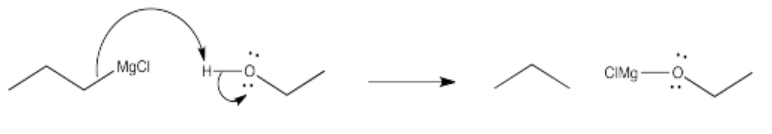Figure $$\PageIndex{30}$$:Figure $$\PageIndex{31}$$:Figure $$\PageIndex{32}$$:

Problem CO11.5.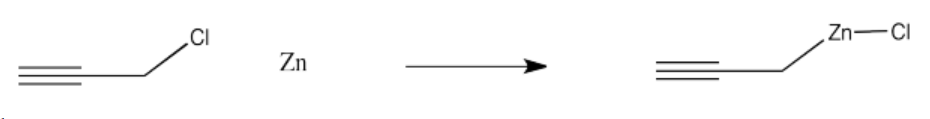Figure $$\PageIndex{33}$$:Figure $$\PageIndex{34}$$:Figure $$\PageIndex{35}$$: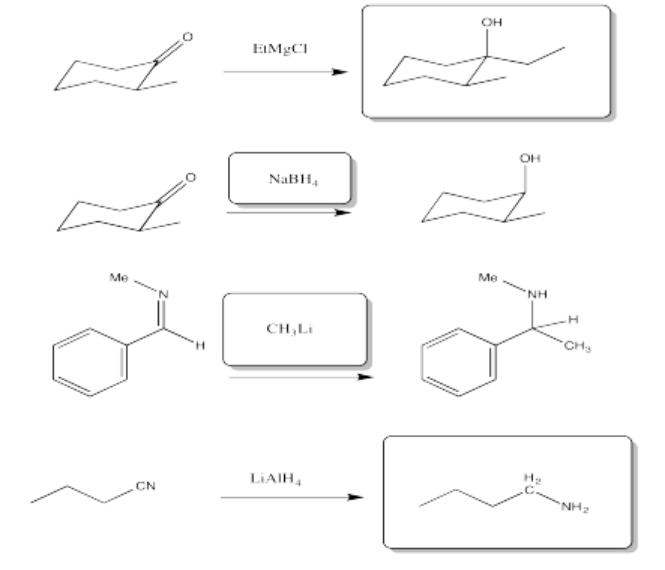Figure $$\PageIndex{36}$$:Figure $$\PageIndex{37}$$:Figure $$\PageIndex{38}$$:Figure $$\PageIndex{39}$$:

a) pentanol b) 2-butanol c) 4-octanol

d) 2-methylpropan-2-ol or 2-methyl-2-pronanol e) 3-methylhexan-2-ol or 3-methyl-3-hexanol

f) 5,6-dimethylheptan-1-ol or 5,6-dimethyl-1-heptanolFigure $$\PageIndex{40}$$:Figure $$\PageIndex{41}$$:Figure $$\PageIndex{42}$$:Figure $$\PageIndex{43}$$:Figure $$\PageIndex{44}$$:Figure $$\PageIndex{45}$$:Figure $$\PageIndex{46}$$:Figure $$\PageIndex{47}$$:Figure $$\PageIndex{48}$$:

Some of these compounds do not have alpha-protons, so they cannot form enolate ions.Figure $$\PageIndex{49}$$:

Problem CO12.11.Figure $$\PageIndex{50}$$:Figure $$\PageIndex{51}$$:Figure $$\PageIndex{52}$$:Figure $$\PageIndex{53}$$:Figure $$\PageIndex{54}$$:Figure $$\PageIndex{55}$$:Figure $$\PageIndex{56}$$: